# GPS Solar Tracker (2)

## MTM Scientific, Inc.

This series of webpages describes a GPS enabled solar tracker based upon the STMAX tilt-tilt platform design. The STMAX solar tracker controller is described in much more detail in our book "Build a Solar Tracker" and at http://www.mtmscientific.com/stmax.html.

The first webpage ( Page 1: GPS Data Acquisition ) of this series described how to acquire time and position data from a GPS module. This webpage describes how to use the acquired GPS information to calculate the position of the sun in the sky. Our final webpage on this topic describes how to use the MEMS ADC counts to aim the tracker: ( Page 3:How to Aim ) The calculation method used here is an adaptation of a software program written by David Williams. The original program was written for generic BASIC, and calculates the Elevation and Azimuth of the sun based upon the observer's position and time. We find it quite remarkable that such a relatively simple program is able to perform this calculation so well. Here is the original program souce code from David Williams: sunalign.bas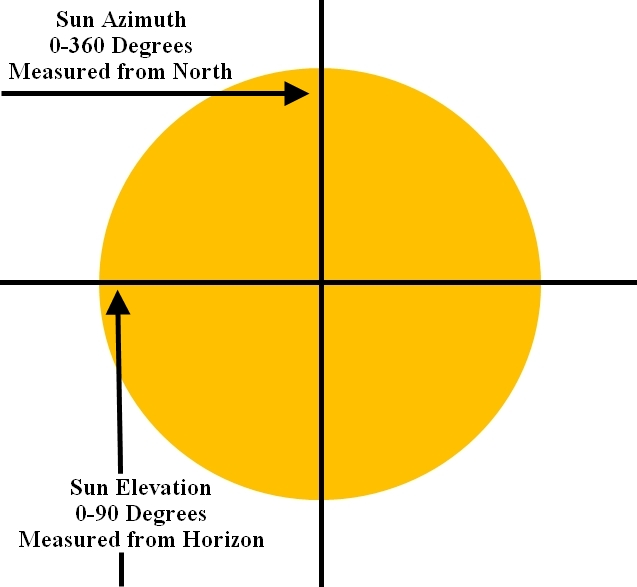Figure 1. Definition of Elevation and Azimuth

You can run the original program using many different versions of BASIC, for example Microsoft's QuickBASIC Version 4.50. The original program is interactive, and will ask for the time, date and location coordinates it needs. We suggest that you familiarize yourself with the original program to gain a basic understanding of how it works.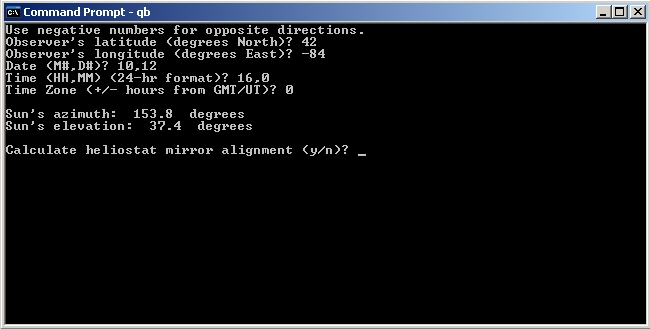Figure 2. Screenshot showing original program being used in QuickBASIC

There are several substantial programming challenges involved in adapting the original program to Picaxe BASIC language, as used by the Picaxe-20X2 microcontroller. The main challenges are: 1) Picaxe BASIC performs all math calculations using only positive single or double byte integers, 2) The Picaxe BASIC trigonometric functions use units of degrees (instead of radians), and 3) The devices using Picaxe BASIC have limited memory space for variables and data. Given these limitations you might be surprised to know this calculation is even possible using a Picaxe-20X2!

Since Picaxe BASIC only performs positive integer math, it is necessary to write code with each variable assigned a bit flag to indicate the sign. Subsequent math operations use the flags in logical branches for calculations. In essence we are creating a method to handle negative and positive integer numbers. The fact that we must perform math using integers requires keeping the arithmetic range between 0 and 255 for a byte variable, or 0 and 65535 for  a word variable.

The limited memory space of devices used with Picaxe BASIC requires the careful assignment and use of variables. The single most important factor in that regard is to use (and reuse) scratchpad variables as often as possible during the calculations. We emphasize this is in the code by often naming those variables as temporary.

Because negative integers are not allowed in Picaxe BASIC we use a method of addition and substraction in several places in the code called 'midpoint math'. Essentially this is a simple technique of shifting the zero origin to the midpoint of a word variable integer (32768).

We also found an opportunity to improve the accuracy of the Picaxe BASIC sine function near a zero crossing. Picaxe BASIC uses a unique coding system to indicate positive and negative trigonometric values, refer to the manuals for more details.  Improving the accuracy of the trigonometric functions in general might be an opportunity for future work.

The calculation of sun position also requires calculation of an entity called the 'Equation of Time' (EOT). Essentially this is the offset in minutes relating the sun's actual position relative to an average theoretical position. We have modified the original code to use a different method of calculating the EOT. See the comments in the code for a web reference.Figure 3. Graph of Equation of Time (EOT)

This code can be easily tested using the simulation mode of the Picaxe Programming Editor. (You don't even need a Picaxe-20X2 chip!) We've done some basic testing of the code for different times, dates and locations. You may want to do more testing for your location. The setup parameters for the input variables are assigned at the start of the code, and can easily be changed as desired.

Here is the complete circuit diagram for the GPS Solar Tracker. You will see we added two optional features: 1) An LED that illumates at Solar Noon (i.e. Azimuth = 180). We also added a digital I/O for a switch, which can be used to detect wind gusts or for any other purpose you may desire. This design builds nicely on all of our previous solar tracker kits. If you study the software listing, you will also see that we added a Heliostat feature in the code. This was fairly easy to do, and it uses the same method given in the original code as written by David Williams.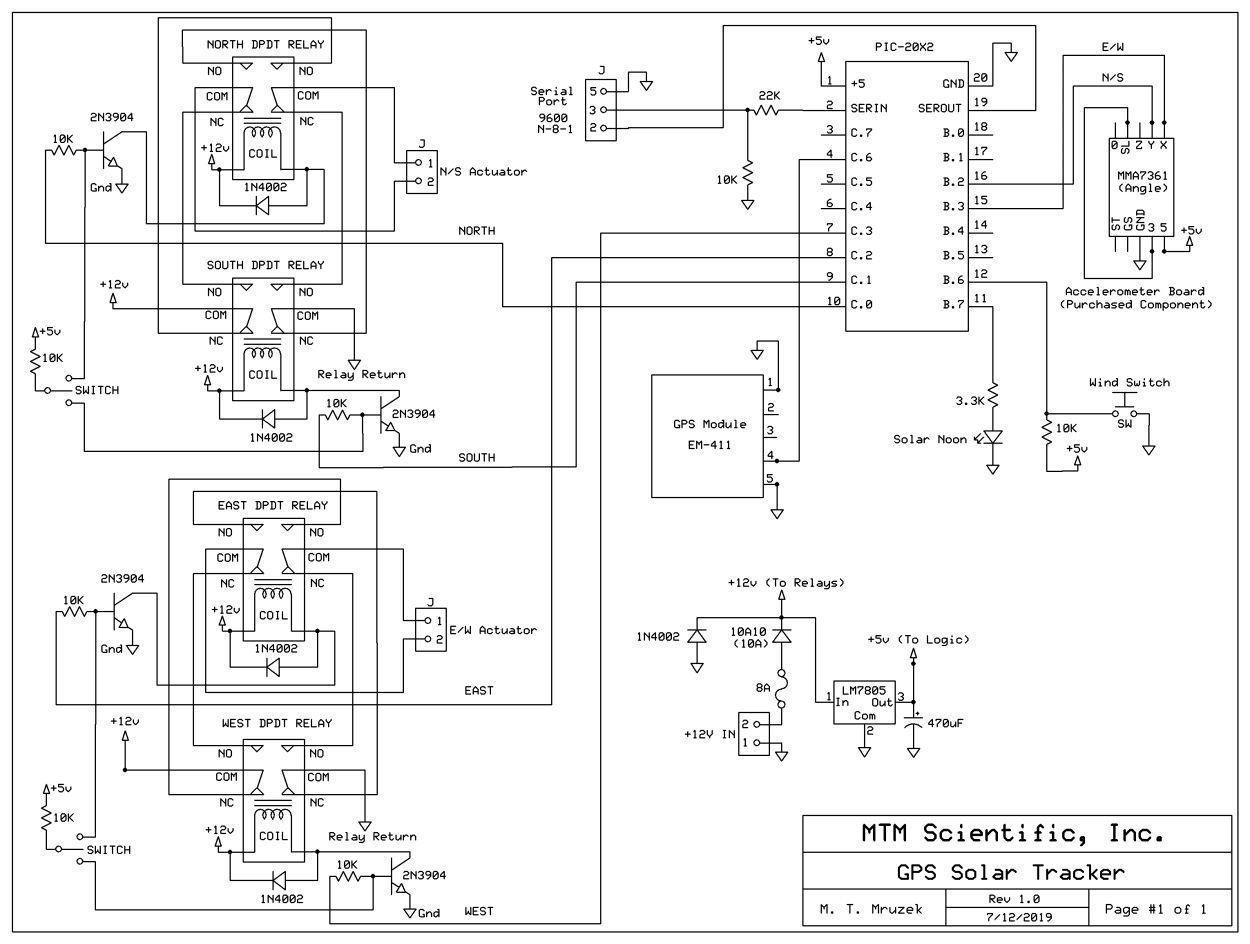Figure 4. Circuit Diagram of GPS Solar Tracker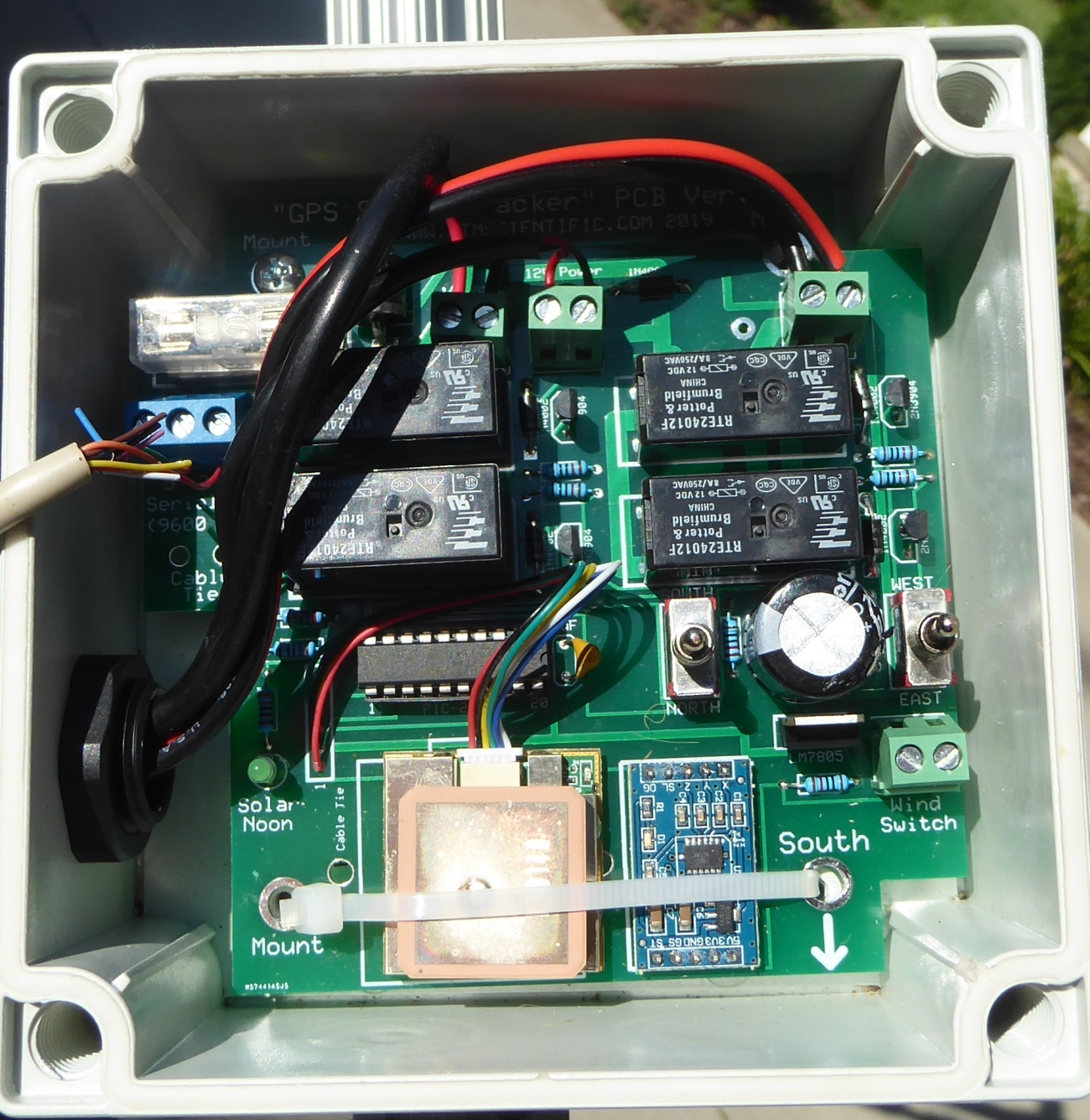Figure 5. GPS Solar Tracker based on STMAX

Here is the Picaxe BASIC code for calculating the position of the sun in the sky based on the observer's location and time. This is the file for download: sun_28.bas

As you can see in the photo, we have designed a PCB for this project. We have some extra PCB's from our prototype run, so if you are interested in duplicating this projecet please write to us by email if you are interested in purchasing one.  This is a really fun project to experiment with! You can populate this board using the parts from out STMAX kit, all you need to add is the GPS module.

The STMAX series of solar trackers use a tilt-tilt platform mechanical design, as detailed in our book. Most of the examples we have shown for tilt-tilt platforms have used the 80-20 aluminum extrusion as the structural element. There are other methods to design tilt-tilt platforms. The photograph in Figure 6 shows a tilt-tilt platform build using 2 gearmotors. The motors are arranged such that one motor does the North-South motion, and the other motor does the East-West motion. The motors were chose to have low RPM output. This type of motor is called a gearhead motor. The GPS Solar Tracker controller rides on the final output stage.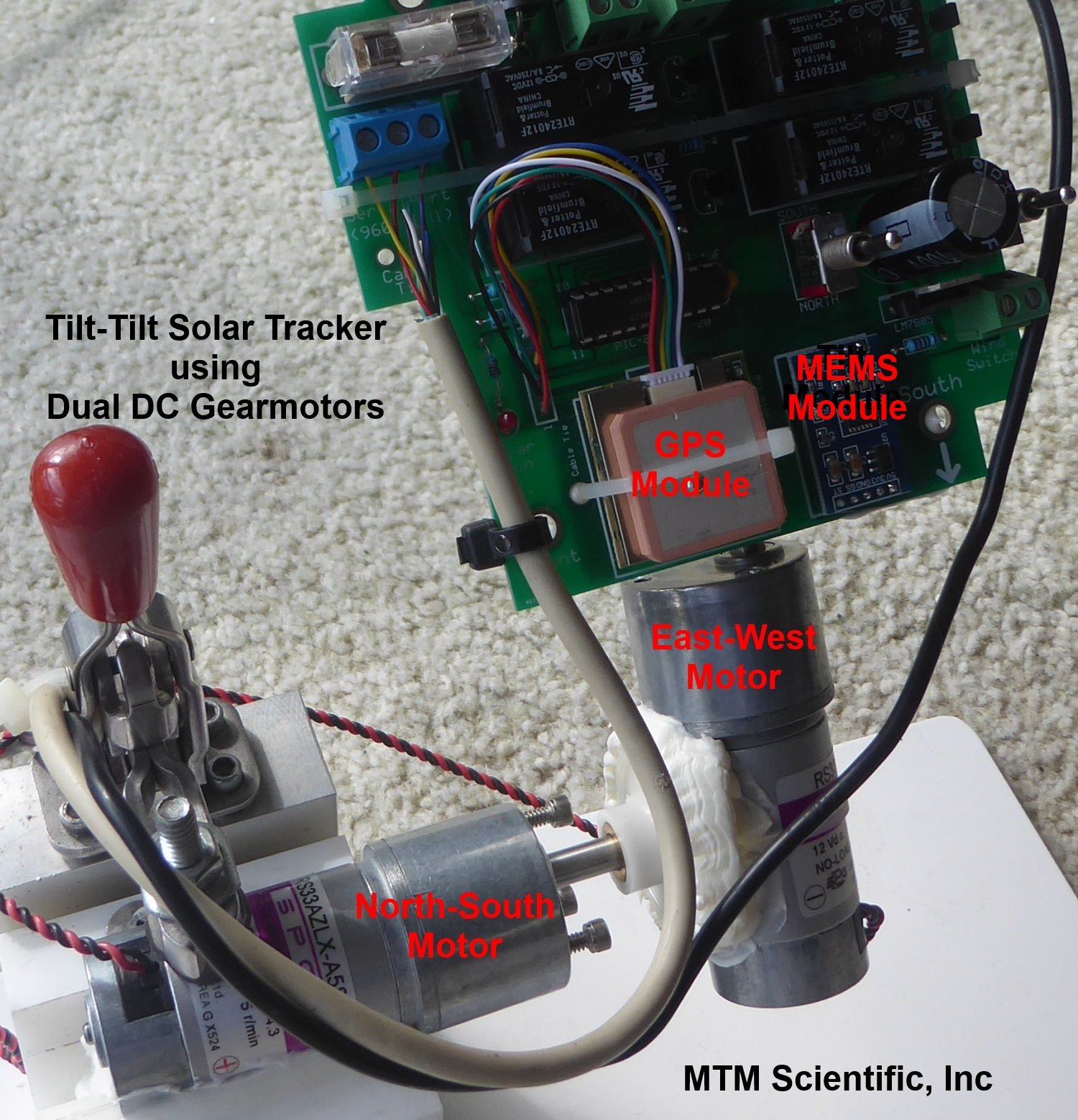Figure 6. Gearhead DC motors used to create a Tilt-Tilt platform